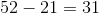# High School Math : Understanding Quartiles and Percentiles

## Example Questions

### Example Question #1 : How To Find The Percentiles For A Data Set

The 70th and 80th percentiles of a set of scores are 78 and 86, respectively; the interquartile range of the scores is 41. Which of these scores is more likely than the others to be at the 25th percentile?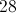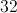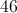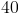Explanation:

The interquartile range of a set of scores is the difference between the third and first quartile - that is, the difference between the 75th and 25th percentiles. The 75th percentile is between 78 and 86, so, if 41 is subtracted from those numbers, the upper and lower bounds of the 25th percentile can be found.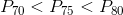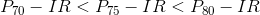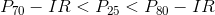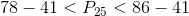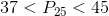Of our choices, only 40 falls in this range.

### Example Question #35 : Basic Statistics

What is the probability of getting a sum of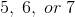when rolling two six-sided fair dice?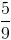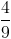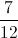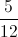Explanation:

The sample space, or total possible outcomes, when rolling two six-sided dice is.

Ways to get what you want: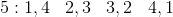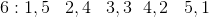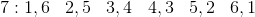So there areways to get a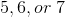.

So the probability becomes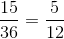### Example Question #36 : Basic Statistics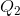is the median of the overall data set.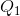is the median of the lower half of the data set.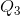is the median of the upper half of the data set.

Find the interquartile range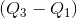for the following data set: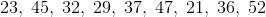Explanation:

First, put the data set in order from smallest to largest: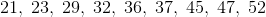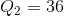which is the middle number in the data set.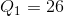which is the average of the two middle numbers of the lower half of the data set.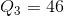which is the average of the two middle numbers of the upper half of the data set.

Thus the interquartile range is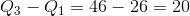Note, the range of the data set is given by the largest number minus the smallest number, or